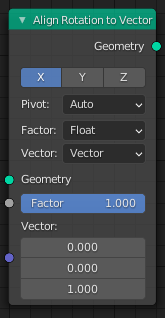# Align Rotation to Vector¶The Align Rotation to Vector node.

The Align Rotation to Vector node rotates points into the given direction. It does so by modifying the Rotation attribute.

## Inputs¶

Geometry

Standard geometry input.

Factor

Determines how much the points are rotated towards the vector. Zero effectively disables the node and one means that the points are aligned with the vector perfectly.

Vector

The direction vector that points should be rotated to. The vector is in the local space of the object that is being modified. When it is all zeros for a point, it is not rotated at all.

## Properties¶

Axis

Local axis of the object that is to be rotated towards the vector input.

Pivot

The local axis to rotate around.

Auto

The best rotation angle is computed automatically. This minimizes the angle of rotation.

X, Y, Z

Rotate around a specific local axis.

Factor

Type of the Factor input socket.

Vector

Type of the Vector input socket.

## Outputs¶

Geometry

Standard geometry output.